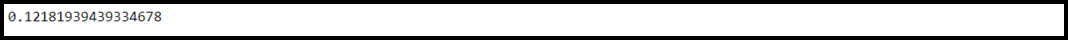# How to Calculate Cramer’s V in Python?

• Last Updated : 28 Feb, 2022

Cramer’s V: It is defined as the measurement of length between two given nominal variables. A nominal variable is a type of data measurement scale that is used to categorize the different types of data. Cramer’s V lies between 0 and 1 (inclusive). 0 indicates that the two variables are not linked by any relation. 1 indicates that there exists a strong association between the two variables. Cramer’s V can be calculated by using the below formula:

√(X2/N) / min(C-1, R-1)

Here,

• X2: It is the Chi-square statistic
• N: It represents the total sample size
• R: It is equal to the number of rows
• C: It is equal to the number of columns

Example 1:

Let us calculate Cramer’s V for a 3 × 3 Table.

## Python3

 `# Load necessary packages and functions``import` `scipy.stats as stats``import` `numpy as np`` ` `# Make a 3 x 3 table``dataset ``=` `np.array([[``13``, ``17``, ``11``], [``4``, ``6``, ``9``],``                    ``[``20``, ``31``, ``42``]])`` ` `# Finding Chi-squared test statistic,``# sample size, and minimum of rows``# and columns``X2 ``=` `stats.chi2_contingency(dataset, correction``=``False``)[``0``]``N ``=` `np.``sum``(dataset)``minimum_dimension ``=` `min``(dataset.shape)``-``1`` ` `# Calculate Cramer's V``result ``=` `np.sqrt((X2``/``N) ``/` `minimum_dimension)`` ` `# Print the result``print``(result)`

Output:Output

The Cramers V comes out to be equal to 0.121 which clearly depicts the weak association between the two variables in the table.

Example 2:

We will now calculate Cramer’s V for larger tables and having unequal dimensions. The Cramers V comes out to be equal to 0.12 which clearly depicts the weak association between the two variables in the table.

## Python3

 `# Load necessary packages and functions``import` `scipy.stats as stats``import` `numpy as np`` ` `# Make a 5 x 4 table``dataset ``=` `np.array([[``4``, ``13``, ``17``, ``11``], [``4``, ``6``, ``9``, ``12``],``                    ``[``2``, ``7``, ``4``, ``2``], [``5``, ``13``, ``10``, ``12``],``                    ``[``5``, ``6``, ``14``, ``12``]])`` ` `# Finding Chi-squared test statistic, ``# sample size, and minimum of rows and``# columns``X2 ``=` `stats.chi2_contingency(dataset, correction``=``False``)[``0``]``N ``=` `np.``sum``(dataset)``minimum_dimension ``=` `min``(dataset.shape)``-``1`` ` `# Calculate Cramer's V``result ``=` `np.sqrt((X2``/``N) ``/` `minimum_dimension)`` ` `# Print the result``print``(result)`

Output:Output

The Cramers V comes out to be equal to 0.146 which clearly depicts the weak association between the two variables in the table.

My Personal Notes arrow_drop_up